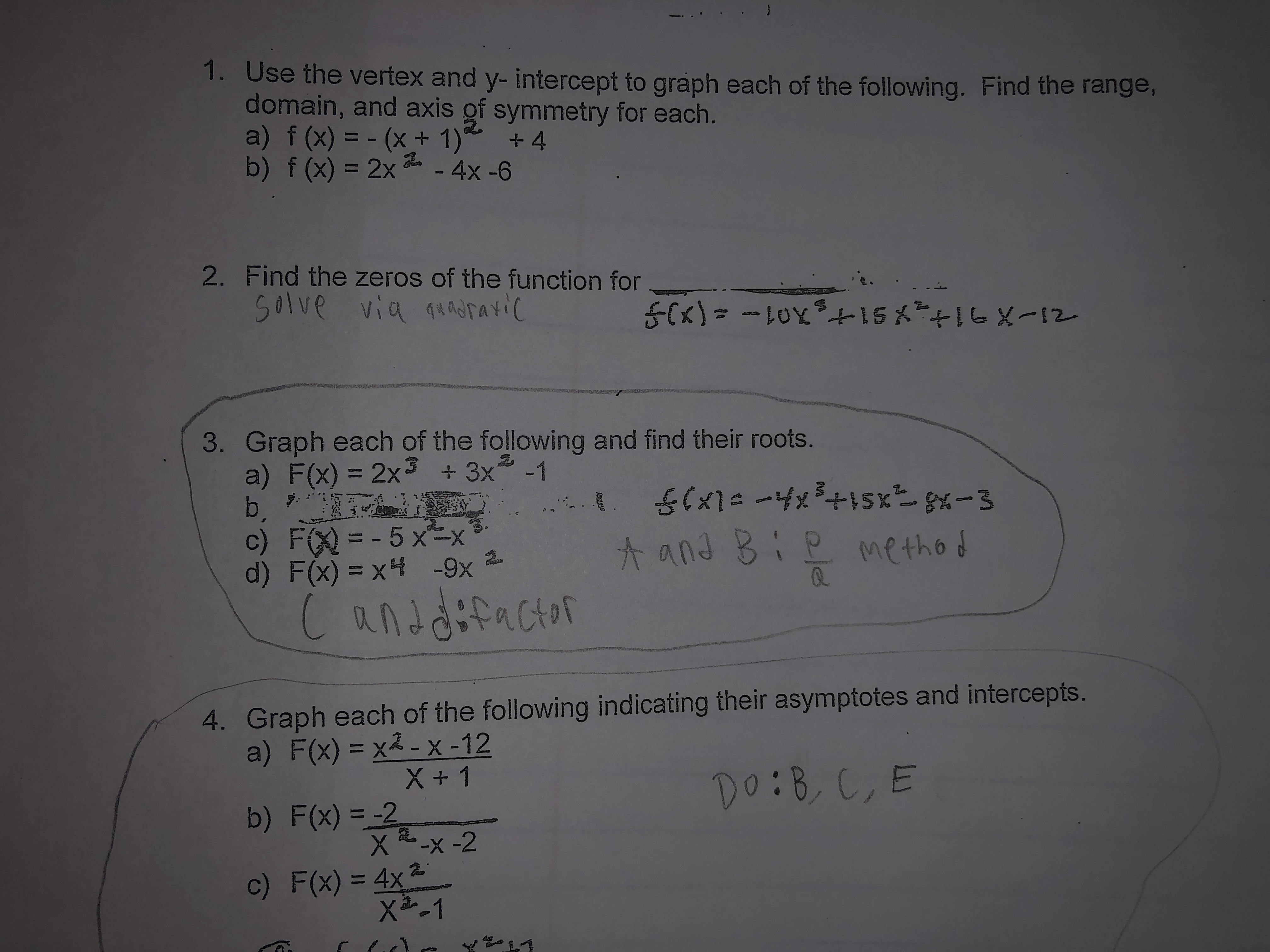# 1. Use the vertex and y- intercept to graph each of the following. Find the range,domain, and axis of symmetry for each.a) f (x) = - (x+1)*b) f (x) = 2x 2 - 4x -6%3D+ 42. Find the zeros of the function forSolve via quadrayic+16X-123. Graph each of the following and find their roots.a) F(x) = 2x3 + 3x -1b. *c) F(X) = - 5 x=x³-d) F(x) = x4 -9x%3DmethodCanddifactor4. Graph each of the following indicating their asymptotes and intercepts.a) F(x) = x2 - X -12X + 1b) F(x) = -2X-x -2Do:B, C,Ec) F(x) = 4x2X2-145

Question
11 viewshelp_outlineImage Transcriptionclose1. Use the vertex and y- intercept to graph each of the following. Find the range, domain, and axis of symmetry for each. a) f (x) = - (x+1)* b) f (x) = 2x 2 - 4x -6 %3D + 4 2. Find the zeros of the function for Solve via quadrayic +16X-12 3. Graph each of the following and find their roots. a) F(x) = 2x3 + 3x -1 b. * c) F(X) = - 5 x=x³- d) F(x) = x4 -9x %3D method Canddifactor 4. Graph each of the following indicating their asymptotes and intercepts. a) F(x) = x2 - X -12 X + 1 b) F(x) = -2 X-x -2 Do:B, C,E c) F(x) = 4x2 X2-1 45 fullscreen
check_circle

Step 1

Part a)

Given:

Step 2

Calculation:

To find domain and range:

Domain:

Step 3

Step 2 as fo...

### Want to see the full answer?

See Solution

#### Want to see this answer and more?

Solutions are written by subject experts who are available 24/7. Questions are typically answered within 1 hour.*

See Solution
*Response times may vary by subject and question.
Tagged in

### Other